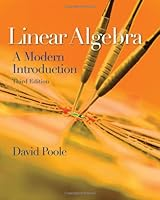# Linear Algebra: A Modern Introduction, 3rd Edition58 Reviews
2010-05-25
768 pages

## Book Description

David Poole's innovative book prepares students to make the transition from the computational aspects of the course to the theoretical by emphasizing vectors and geometric intuition from the start. Designed for a one- or two-semester introductory course and written in simple, " English" the book presents interesting examples before abstraction. This immediately follows up theoretical discussion with further examples and a variety of applications drawn from a number of disciplines, which reinforces the practical utility of the math, and helps students from a variety of backgrounds and learning styles stay connected to the concepts they are learning. Poole's approach helps students succeed in this course by learning vectors and vector geometry first in order to visualize and understand the meaning of the calculations that they will encounter and develop maturity for thinking abstractly.

Ch 1: Vectors
Ch 2: of Linear Equations
Ch 3: Matrices
Ch 4: Eigenvalues and Eigenvectors
Ch 5: Orthogonality
Ch 6: Vector Spaces
Ch 7: Distance and Approximation
Appendix A: Mathematical Notation and Methods of Proof
Appendix B: Mathematical Induction
Appendix C: Complex Numbers
Appendix D: Polynomials

## Book Details

• Title: Linear Algebra: A Modern Introduction, 3rd Edition
• Author:
• Length: 768 pages
• Edition: 3
• Language: English
• Publisher:
• Publication Date: 2010-05-25
• ISBN-10: 0538735457
• ISBN-13: 9780538735452